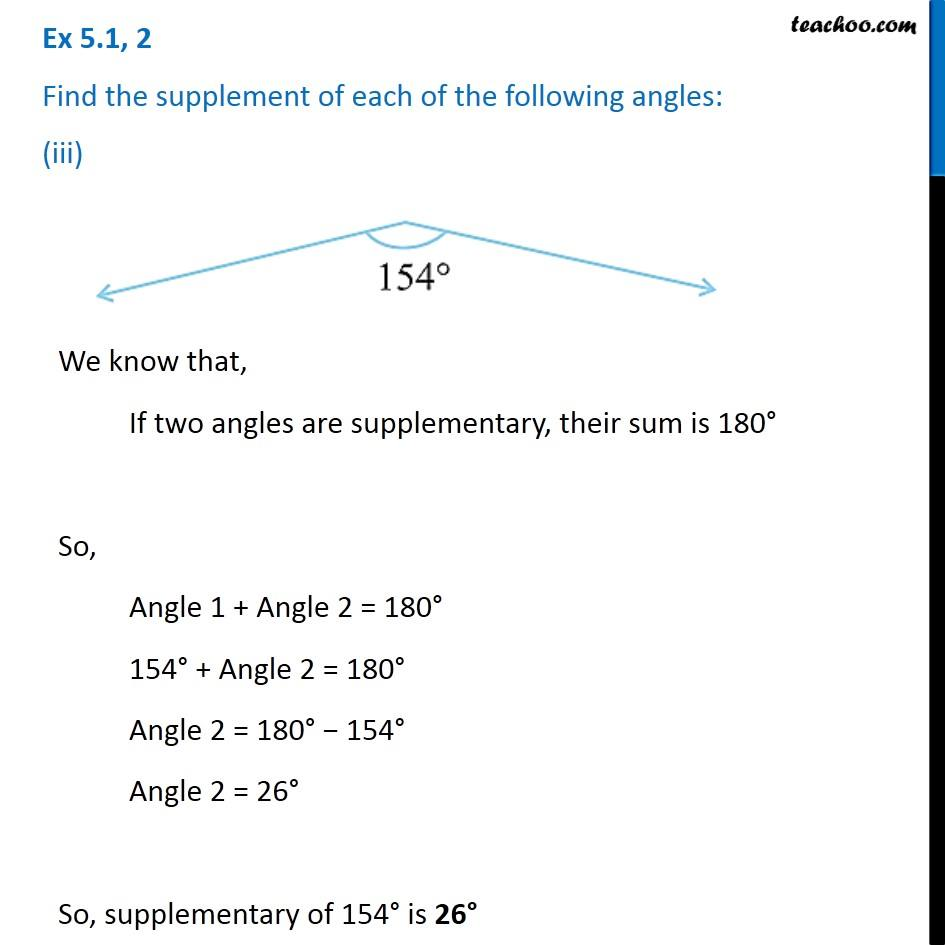Ex 5.1

Chapter 5 Class 7 Lines and Angles
Serial order wise### Transcript

Ex 5.1, 2 Find the supplement of each of the following angles: (iii) We know that, If two angles are supplementary, their sum is 180° So, Angle 1 + Angle 2 = 180° 154° + Angle 2 = 180° Angle 2 = 180° − 154° Angle 2 = 26° So, supplementary of 154° is 26°